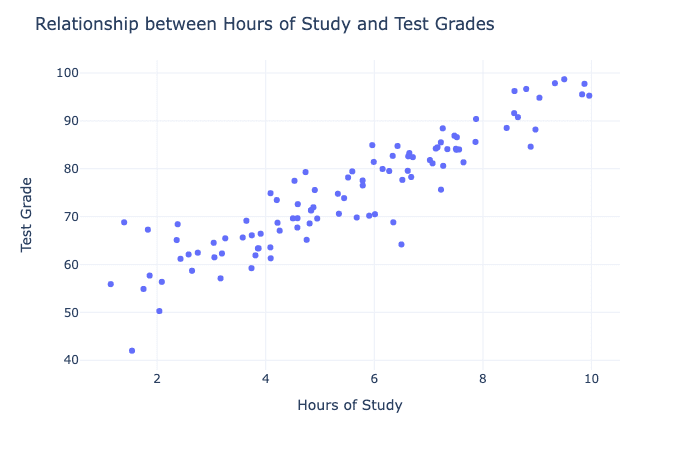# What is the difference between independent and dependent variables?###### Author

📊🤔🔍

Hey, guys! Today we’re talking about the relationship between variables. 📈

When exploring the relationship between variables, it’s important to think about how one variable might be causing the other. This is because it can help you identify possible causal relationships and make more accurate predictions about future outcomes. 🔍

For example, let’s say you’re exploring the relationship between exercise and weight loss. By thinking about how exercise might be causing weight loss, you can design a better exercise program that is more likely to lead to weight loss. 🏋️‍♀️🩸

What is the difference between an independent variable and a dependent variable? 🤔

An independent variable is a variable that is manipulated or changed by the researcher. A dependent variable is a variable that is measured or observed and is affected by the independent variable. 📊For example, let’s say you’re looking at a scatterplot that shows the relationship between the number of hours of study and the grade on a math test. The number of hours of study would be the independent variable, because it’s the variable that is being manipulated (i.e., students can choose to study more or less). The grade on the math test would be the dependent variable, because it’s the variable that is being observed and affected by the independent variable (i.e., the number of hours of study may affect the grade on the math test). 📈

So there you have it, guys! When exploring the relationship between variables, it’s important to think about how one variable might be causing the other. An independent variable is a variable that is manipulated, while a dependent variable is a variable that is observed and affected by the independent variable. 🤓📊🔍